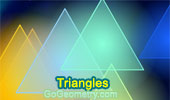# Triangles: Theorems and Problems 30Proposed Problem 127. Centroid and Incenter of a Triangle, Parallel, Proportions.

Proposed Problem 126. Incenter of Triangle, Angle Bisector, Proportions.

The significance of the Pythagorean theorem  by Jacob Bronowski.
Pythagorean Theorem, 47th Proposition of Euclid's Book I.

Proposed Problem 125. Area of Triangle, Star, Trisection of Sides.

Proposed Problem 124. Area of triangle, Similarity, Trisection of Sides.

Proposed Problem 123. Area of triangle, Similarity, Trisection of Sides.

Proposed Problem 122. Marion Walter's Theorem. Area of triangle and Hexagon, Trisection of Sides.

Proposed Problem 121. Similarity and Area of triangle, Trisection of Sides.

Bottema's Theorem:
Triangle and Squares with Interactive Geometry Software
Step-by-Step construction, Manipulation, and animation.